# 13.Calculate the potential (E) at 25°C for the following cell. ICu2 (0.024 M)Ag (0.0048 M) Cu2+...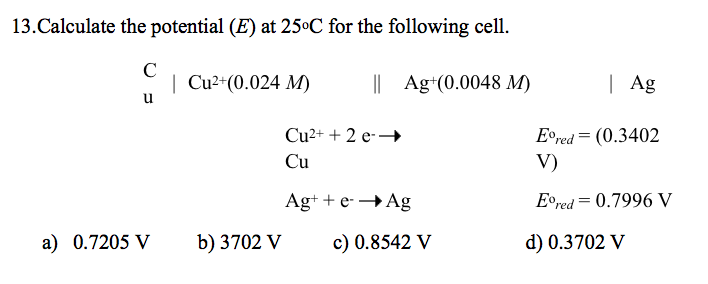13.Calculate the potential (E) at 25°C for the following cell. ICu2 (0.024 M)Ag (0.0048 M) Cu2+ + 2 e-? Cu Eored (0.3402 Agt te-Ag E-red = 0.7996 V a) 0.7205 V b) 3702V c 0.8542 V d) 0.3702 V

Option D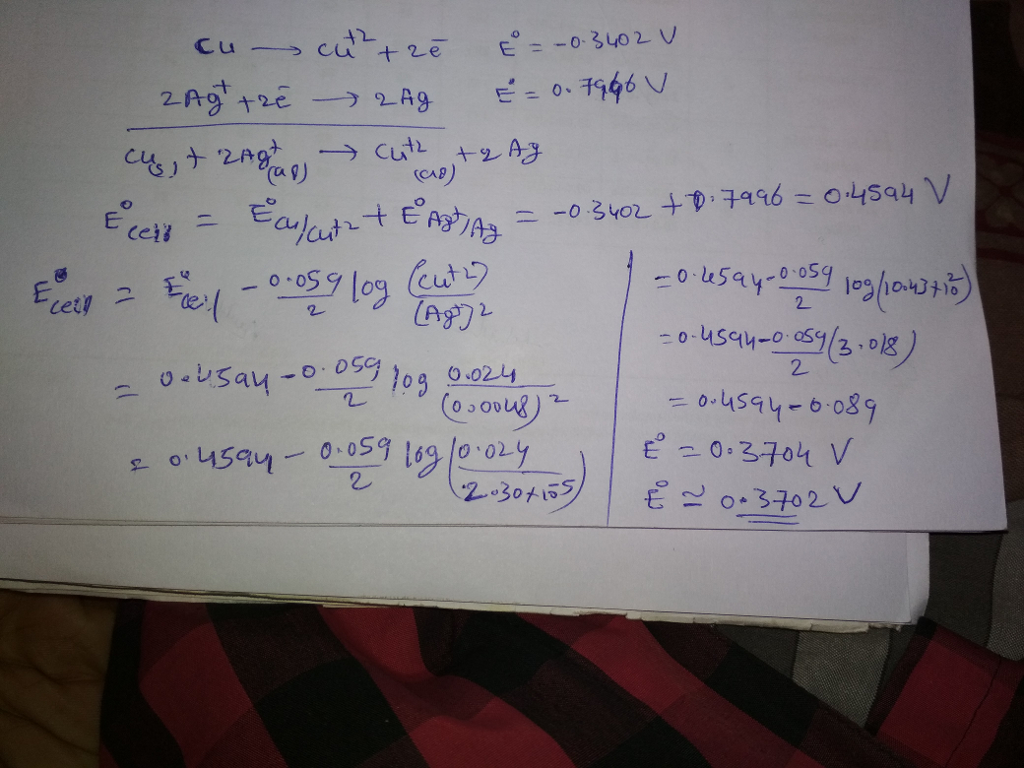#### Earn Coin

Coins can be redeemed for fabulous gifts.

Similar Homework Help Questions
• ### at 25 degrees celcius E cell Determining 1. Cul Cu2+ 11 Agt (Ag 1.0 M Cu,...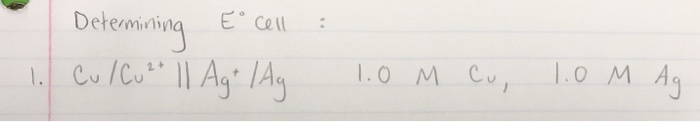at 25 degrees celcius E cell Determining 1. Cul Cu2+ 11 Agt (Ag 1.0 M Cu, 1.0 M Ag

• ### Calculate the cell potential for the reaction below when the concentrations of ions are: [Ag+ ]...

Calculate the cell potential for the reaction below when the concentrations of ions are: [Ag+ ] = 0.010 M and [Cu2+] = 0.750 M, at 25 °C. Cu(s) + 2Ag+ (aq) --> Cu2+(aq) + 2Ag(s) Given the standard reduction potentials: Cu2+(aq) + 2e– → Cu(s) Eϴ = 0.34 V Ag+ (aq) + e– → Ag(s) Eϴ = 0.80 V (A) 0.35 V (B) 0.44 V (C) 0.46 V (D) 0.48 V (E) 0.57 V

• ### Calculate the E°cell for the following reaction:                 Cu2+ (aq) + Ni (s) → Cu (s)...

Calculate the E°cell for the following reaction:                 Cu2+ (aq) + Ni (s) → Cu (s) + Ni2+ (aq) A) (-0.59 ± 0.01) V B) (-0.09 ± 0.01) V C) (0.59 ± 0.01) V D) (0.09 ± 0.01) V --------------------------------------------------------------------------------------------------------- What is the proper line notation for the following reaction? Cu (s) + 2Ag+ (aq) → Cu2+ (aq) + 2Ag (s) A) Cu2+ | Cu || Ag | Ag+ B) Ag+ | Ag || Cu | Cu2+ C) Cu |...

• ### Calculate the E°cell for the following reaction:                 Cu2+ (aq) + Ni (s) → Cu (s)...

Calculate the E°cell for the following reaction:                 Cu2+ (aq) + Ni (s) → Cu (s) + Ni2+ (aq) A) (-0.59 ± 0.01) V B) (-0.09 ± 0.01) V C) (0.59 ± 0.01) V D) (0.09 ± 0.01) V --------------------------------------------------------------------------------------------------------- What is the proper line notation for the following reaction? Cu (s) + 2Ag+ (aq) → Cu2+ (aq) + 2Ag (s) A) Cu2+ | Cu || Ag | Ag+ B) Ag+ | Ag || Cu | Cu2+ C) Cu |...

• ### Question 2 Consider a galvanic cell with the following cell notation: Cu(s)|Cu2+(0.0200 M) || Ag+ (0.0200...

Question 2 Consider a galvanic cell with the following cell notation: Cu(s)|Cu2+(0.0200 M) || Ag+ (0.0200 M)|Ag(s) a) Calculate the electrode potential at the cathode (Ecathode) b) Calculate the electrode potential at the anode (Eanode) c) Calculate the cell potential (Ecell)

• ### Calculate the potential of the electrochemical cell and determine if it is spontaneous as written at 25 °C Cu(s) Cu2 (0...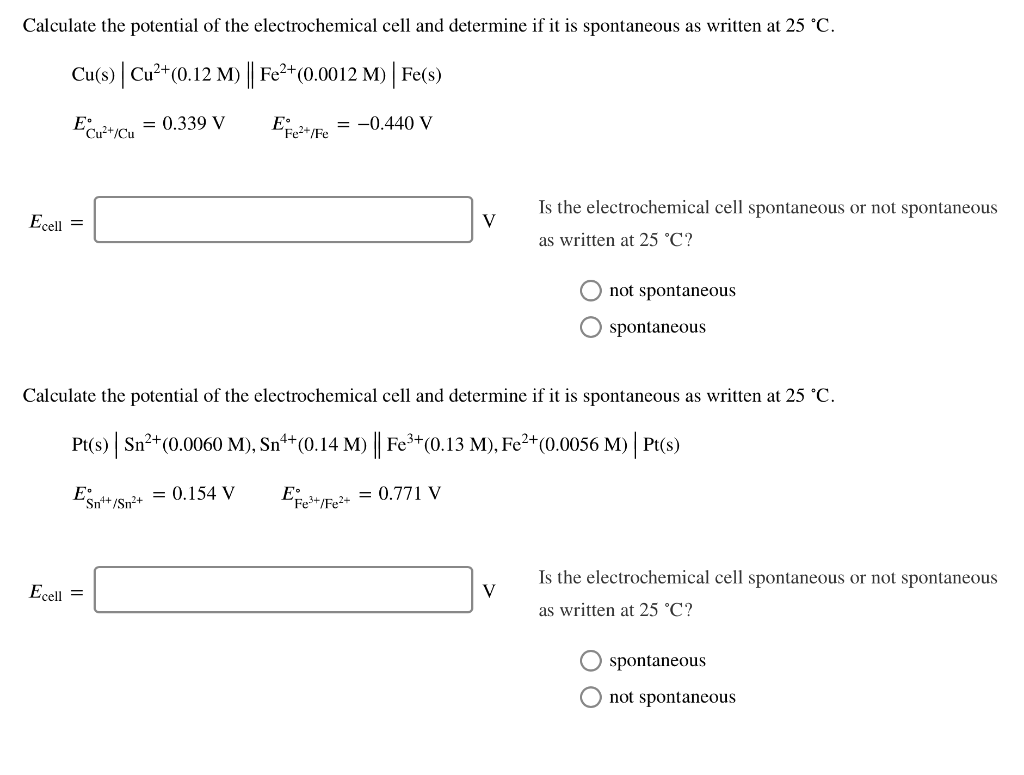Calculate the potential of the electrochemical cell and determine if it is spontaneous as written at 25 °C Cu(s) Cu2 (0.12 M |Fe2 (0.0012 M) Fe(s) E2 =-0.440 V Efe/Fe = 0.339 V Cu2t/Cu Is the electrochemical cell spontaneous or not spontaneous Ecell V as written at 25 °C? not spontaneous spontaneous Calculate the potential of the electrochemical cell and determine if it is spontaneous as written at 25 °C. Pt(s) Sn2(0.0060 M), Sn4+(0.14 M) Fe3+(0.13 M), Fe2+(0.0056 M) Pt(s)...

• ### A voltaic cell consists of a Pb/Pb2+ half-cell and a Cu/Cu2+ half-cell at 25 oC. The...

A voltaic cell consists of a Pb/Pb2+ half-cell and a Cu/Cu2+ half-cell at 25 oC. The initial concentrations of Pb2+ and Cu2+ are 0.0500 M and 1.50 M, respectively. What are the concentrations of Pb2+ and Cu2+ when the cell potential falls to 0.370 V? Cu2+(ag) + Pb(s) → Cu(s) + Pb2+(ag)        Hint: [Pb2+] + [Cu2+] = (1.5M + 0.05M) = 1.55 M total Hint: the standard potential of Pb2+ + 2e-   → Pb(s) is -0.130 and the standard potential...

• ### Calculate the cell potential, E, for the given reactions at 25.00 °C using the ion concentrations...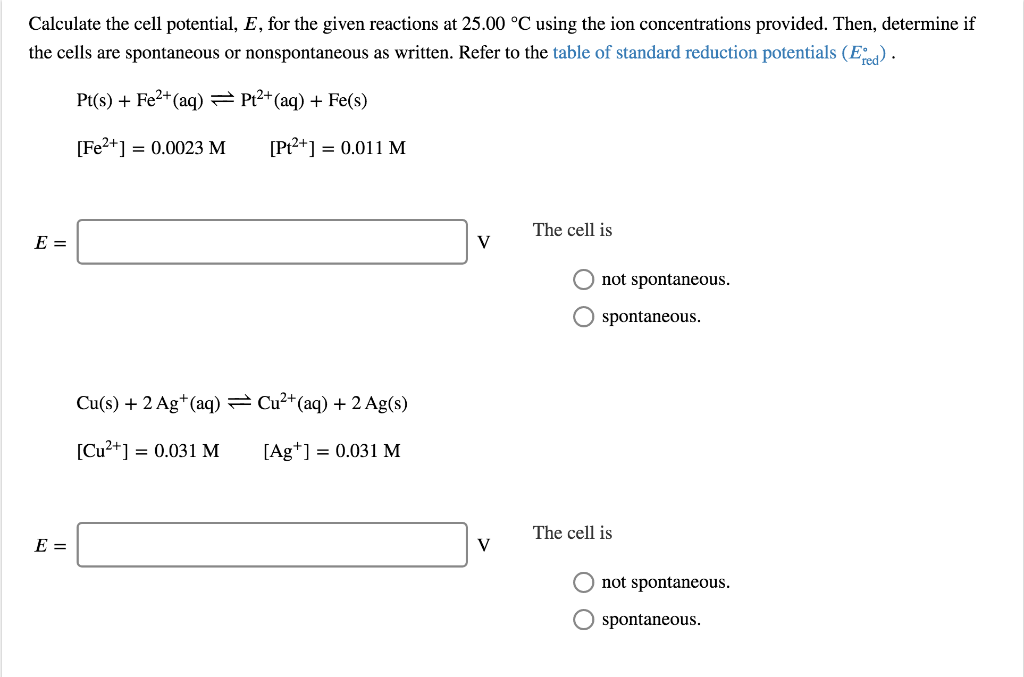Calculate the cell potential, E, for the given reactions at 25.00 °C using the ion concentrations provided. Then, determine if the cells are spontaneous or nonspontaneous as written. Refer to the table of standard reduction potentials (E Pt(s)Fe2+(aq) Pt2+(aq) + Fe(s) [Fe2+ [Pt2+] = 0.0023 M = 0.011 M The cell is V E = O not spontaneous. O spontaneous Cu(s)2 Ag (aq) Cu2+(aq) + 2 Ag(s) [Cu2+0.031 M [Ag*] = 0.031 M The cell is V E = O...

• ### Calculate the cell potential for the following cell at 25 ° C Cd (s) | Cd...

Calculate the cell potential for the following cell at 25 ° C Cd (s) | Cd (NO3) 2 (aq, 0.010 M) || KBr (aq, 0.050 M), Ag + (aq) | AgBr (s) | Ag (s) using normal potentials E⦵ (Cd 2+ / Cd) = -0.40 V and E⦵ (Ag + / Ag) = 0.80 V, and the solubility of AgBr, which is 7.7 · 10-13.

• ### Calculate the potential of the electrochemical cell and determine if it is spontaneous as written at...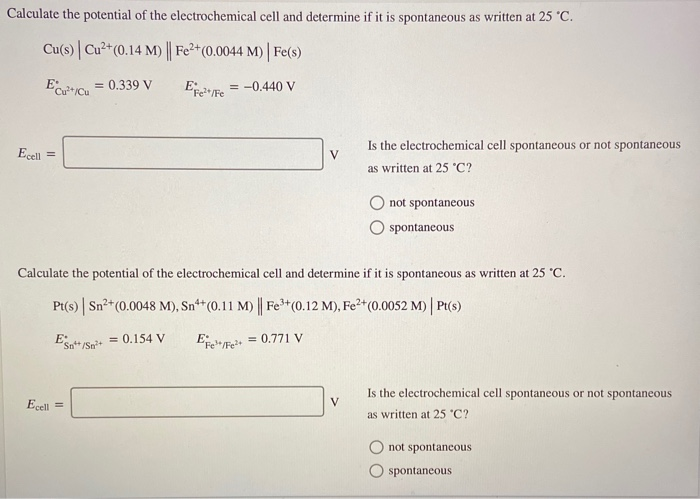Calculate the potential of the electrochemical cell and determine if it is spontaneous as written at 25 °C. Cu(s) Cu2+(0.14 M) | Fe2+(0.0044 M) Fe(s) Ecu?+Icu = 0.339 V Efez lfe = -0.440 V Ecell = v Is the electrochemical cell spontaneous or not spontaneous as written at 25 °C? O not spontaneous O spontaneous Calculate the potential of the electrochemical cell and determine if it is spontaneous as written at 25 °C. Pt(s) Sn2+(0.0048 M), Snº+(0.11 M) || Fe3+(0.12...